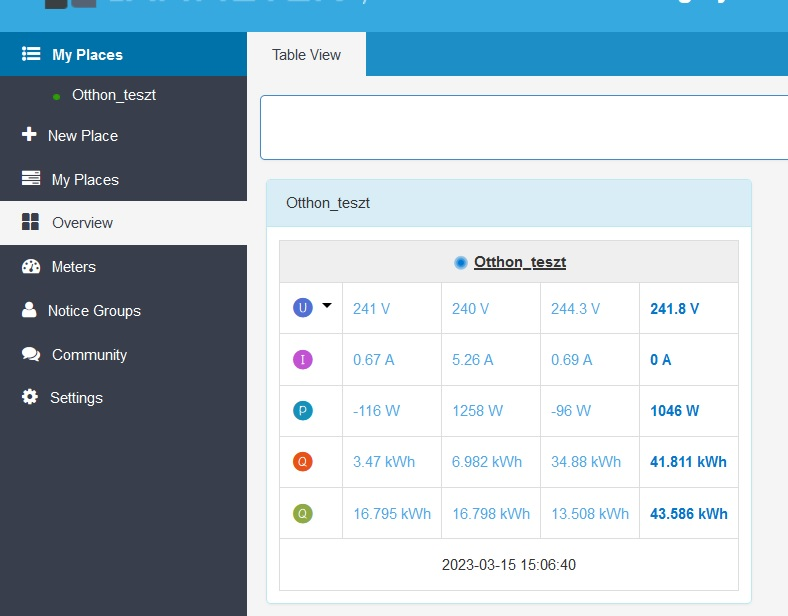### Netmeter - missing current value

Hi,

I have a question;

I don't understand why I see a zero value for the current?

I set up the netmeter function on both the meter and the cloud application.

On local access, the fourth column appeared, but there is also a zero value for the current.

Can you help me, what's wrong?

Thanks,

Csaba1
101
2
0
0

###### Sort replies by:

Hi:

There is no meaning in the current reading value of the fourth column

This is the definition of the fourth column of data

If you open the Net Metring mode(https://www.iammeter.com/docs/net-energy-meter),  it is the NEM measurement result.

Voltage: arithmetic average

Current: no exact meaning, does not mean it is zero value

Power: arithmetic sum power value of the three-phase

kWh: counted in NEM mode.

Otherwise, it is the arithmetic sum result(default: normal mode).

Voltage: arithmetic average

Current: no exact meaning, do not mean it is zero value

Power: arithmetic sum power value of the three-phase

kWh: arithmetic sum of the three-phase

Looks like you are new here. Register for free, learn and contribute.